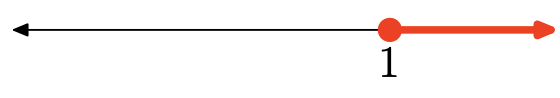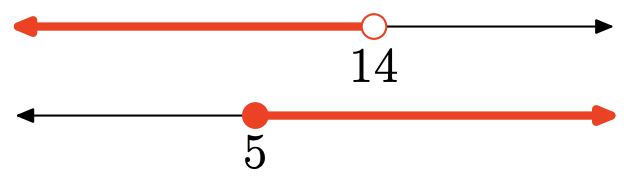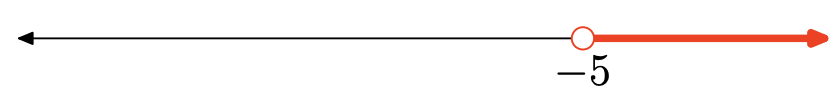# 2.8E: Exercises for Section 2.8

$$\newcommand{\vecs}{\overset { \rightharpoonup} {\mathbf{#1}} }$$ $$\newcommand{\vecd}{\overset{-\!-\!\rightharpoonup}{\vphantom{a}\smash {#1}}}$$$$\newcommand{\id}{\mathrm{id}}$$ $$\newcommand{\Span}{\mathrm{span}}$$ $$\newcommand{\kernel}{\mathrm{null}\,}$$ $$\newcommand{\range}{\mathrm{range}\,}$$ $$\newcommand{\RealPart}{\mathrm{Re}}$$ $$\newcommand{\ImaginaryPart}{\mathrm{Im}}$$ $$\newcommand{\Argument}{\mathrm{Arg}}$$ $$\newcommand{\norm}{\| #1 \|}$$ $$\newcommand{\inner}{\langle #1, #2 \rangle}$$ $$\newcommand{\Span}{\mathrm{span}}$$ $$\newcommand{\id}{\mathrm{id}}$$ $$\newcommand{\Span}{\mathrm{span}}$$ $$\newcommand{\kernel}{\mathrm{null}\,}$$ $$\newcommand{\range}{\mathrm{range}\,}$$ $$\newcommand{\RealPart}{\mathrm{Re}}$$ $$\newcommand{\ImaginaryPart}{\mathrm{Im}}$$ $$\newcommand{\Argument}{\mathrm{Arg}}$$ $$\newcommand{\norm}{\| #1 \|}$$ $$\newcommand{\inner}{\langle #1, #2 \rangle}$$ $$\newcommand{\Span}{\mathrm{span}}$$$$\newcommand{\AA}{\unicode[.8,0]{x212B}}$$

In Exercises 1 - 8, use both interval and set-builder notation to describe the intersection of the two intervals shown on the graph. Also, sketch the graph of the intersection on the real number line.

Exercise 1The intersection is the set of points that are in both intervals (shaded on both graphs). Graph of the intersection:Set-Builder Notation: $$\{x \, | \, x \geq 1\}$$

Interval Notation: $$[1, \infty)$$

Exercise 2Exercise 3There are no points that are in both intervals (shaded in both), so there is no intersection. Graph of the intersection:no intersection

Set-Builder Notation: $$\{ }$$

Interval Notation: none

Exercise 4Exercise 5The intersection is the set of points that are in both intervals (shaded in both). Graph of the intersection:Set-Builder Notation: $$\{x \, | \, -6 \leq x \leq 2\}$$

Interval Notation: $$[-6,2]$$

Exercise 6Exercise 7The intersection is the set of points that are in both intervals (shaded in both). Graph of the intersection:Set-Builder Notation: $$\{x \, | \, x \geq 9\}$$

Interval Notation: $$[9, \infty)$$

Exercise 8In Exercises 9 - 16, use both interval notation and set-builder notation to describe the union of the two intervals shown on the graph. Also, sketch the graph of the union on the real number line.

Exercise 9The union is the set of all points that are in one interval or the other (shaded in either graph). Graph of the union:Set-Builder Notation: $$\{x \, | \, x \leq-8\}$$

Interval Notation: $$(-\infty,-8]$$

Exercise 10Exercise 11The union is the set of all points that are in one interval or the other (shaded in either graph). Graph of the union:Set-Builder Notation: $$\{x \, | \, x \leq 9 \text { or } x>15\}$$

Interval Notation: $$(-\infty, 9] \cup(15, \infty)$$

Exercise 12Exercise 13The union is the set of all points that are in one interval or the other (shaded in either). Graph of the union:Set-Builder Notation: $$\{x \, | \, x<3\}$$

Interval Notation: $$(-\infty, 3)$$

Exercise 14Exercise 15The union is the set of all points that are in one interval or the other (shaded in either). Graph of the union:Set-Builder Notation: $$\{x \, | \, x \geq 9\}$$

Interval Notation: $$[9, \infty)$$

Exercise 16In Exercises 17 - 32, use interval notation to describe the given set. Also, sketch the graph of the set on the real number line.

Exercise 17

$$\{x \, | \, x \geq-6 \text { and } x>-5\}$$

This set is the same as $$\{x \, | \, x>-5\}$$, which is $$(-5, \infty)$$ in interval notation. Graph of the set:Exercise 18

$$\{x \, | \, x \leq 6 \text { and } x \geq 4\}$$

Exercise 19

$$\{x \, | \, x \geq-1 \text { or } x<3\}$$

Every real number is in one or the other of the two intervals. Therefore, the set is the set of all real numbers $$(-\infty, \infty)$$. Graph of the set:Exercise 20

$$\{x \, | \, x>-7 \text { and } x>-4\}$$

Exercise 21

$$\{x \, | \, x \geq -1 \text { or } x>6\}$$

This set is the same as $$\{x \, | \, x \geq-1\}$$, which is $$[-1, \infty)$$ in interval notation. Graph of the set:Exercise 22

$$\{x \, | \, x \geq 7 \text { or } x<-2\}$$

Exercise 23

$$\{x \, | \, x \geq 6 \text { or } x>-3\}$$

This set is the same as $$\{x \, | \, x>-3\}$$, which is $$(-3, \infty)$$ in interval notation. Graph of the set:Exercise 24

$$\{x \, | \, x \leq 1 \text { or } x>0\}$$

Exercise 25

$$\{x \, | \, x<2 \text { and } x<-7\}$$

This set is the same as $$\{x \, | \, x<-7\}$$, which is $$(-\infty,-7)$$ in interval notation. Graph of the set:Exercise 26

$$\{x \, | \, x \leq-3 \text { and } x<-5\}$$

Exercise 27

$$\{x \, | \, x \leq-3 \text { or } x \geq 4\}$$

This set is the union of two intervals, $$(-\infty,-3] \cup[4, \infty)$$. Graph of the set:Exercise 28

$$\{x \, | \, x<11 \text { or } x \leq 8\}$$

Exercise 29

$$\{x \, | \, x \geq 5 \text { and } x \leq 1\}$$

There are no numbers that satisfy both inequalities. Thus, there is no intersection. Graph of the set:Exercise 30

$$\{x \, | \, x<5 \text { or } x<10\}$$

Exercise 31

$$\{x \, | \, x \leq 5 \text { and } x \geq-1\}$$

This set is the same as $$\{x \, | \, -1 \leq x \leq 5\}$$, which is [−1, 5] in interval notation. Graph of the setExercise 32

$$\{x \, | \, x>-3 \text { and } x<-6\}$$

In Exercises 33 - 44, solve the inequality. Express your answer in both interval and set-builder notations, and graph the solution on a number line.

Exercise 33

$$-8 x-3 \leq-16 x-1$$

\begin{aligned} & -8 x-3 \leq-16 x-1 \\ \Longrightarrow \quad & − 8x + 16x \leq −1 + 3 \\ \Longrightarrow \quad& 8x \leq 2 \\ \Longrightarrow \quad & x \leq \frac{1}{4}\end{aligned}

Thus, the solution interval isSet-Builder Notation: $$\{x \, | \, x \leq \frac{1}{4}\}$$

Interval Notation: $$(−\infty, \frac{1}{4}]$$

Exercise 34

$$6 x-6>3 x+3$$

Exercise 35

$$-12 x+5 \leq-3 x-4$$

\begin{aligned} & -12 x+5 \leq-3 x-4 \\ \Longrightarrow \quad & -12x + 3x \leq −4 − 5 \\ \Longrightarrow \quad& -9x \leq -9 \\ \Longrightarrow \quad & x \geq 1\end{aligned}

Thus, the solution interval isSet-Builder Notation: $$\\{x \, | \, x\geq 1\}$$

Interval Notation: $$[1,\infty)$$

Exercise 36

$$7 x+3 \leq-2 x-8$$

Exercise 37

$$-11 x-9<-3 x+1$$

\begin{aligned} & − 11x − 9 < −3x + 1 \\ \Longrightarrow \quad & − 11x + 3x < 1 + 9 \\ \Longrightarrow \quad& − 8x < 10 \\ \Longrightarrow \quad & x > -\frac{5}{4}\end{aligned}

Thus, the solution interval isSet-Builder Notation: $$\{x \, | \, x >−\frac{5}{4} \}$$

Interval Notation: $$(−\frac{5}{4} ,\infty)$$

Exercise 38

$$4 x-8 \geq-4 x-5$$

Exercise 39

$$4 x-5>5 x-7$$

\begin{align*} & 4x − 5 > 5x − 7\\ \Longrightarrow \quad & 4x − 5x > −7 + 5 \\ \Longrightarrow \quad& − x > −2 \\ \Longrightarrow \quad &x < 2\end{align*}
Thus, the solution interval isSet-Builder Notation: $$\{x \, | \, x < 2\}$$

Interval Notation: $$(−\infty, 2)$$

Exercise 40

$$-14 x+4>-6 x+8$$

Exercise 41

$$2 x-1>7 x+2$$

\begin{aligned} & 2x − 1 > 7x + 2\\ \Longrightarrow \quad & 2x − 7x > 2 + 1 \\ \Longrightarrow \quad& − 5x > 3 \\ \Longrightarrow \quad &x < −\frac{3}{5}\end{aligned}
Thus, the solution interval isSet-Builder Notation: $$\{x \, | \, x < −\frac{3}{5}\}$$

Interval Notation: $$(−\infty, −\frac{3}{5})$$

Exercise 42

$$-3 x-2>-4 x-9$$

Exercise 43

$$-3 x+3<-11 x-3$$

\begin{aligned} & − 3x + 3 < −11x − 3\\ \Longrightarrow \quad & − 3x + 11x < −3 − 3 \\ \Longrightarrow \quad& 8x < −6 \\ \Longrightarrow \quad &x < -\frac{3}{4}\end{aligned}
Thus, the solution interval isSet-Builder Notation: $$\{x \, | \, x < −\frac{3}{4}\}$$

Interval Notation: $$(−\infty, −\frac{3}{4})$$

Exercise 44

$$6 x+3<8 x+8$$

In Exercises 45-53, solve the compound inequality. Express your answer in both interval and set-builder notations, and graph the solution on a number line.

Exercise 45

$$2 x-1<4$$ or $$7 x+1 \geq-4$$

\begin{aligned} & 2x − 1 < 4 \text{ or } 7x + 1 \geq −4\\ \Longrightarrow \quad & 2x < 5\quad \text{or}\quad 7x \geq −5 \\ \Longrightarrow \quad&x<\frac{5}{2}\quad\text{or}\quad x\geq-\frac{5}{7}\end{aligned}For the union, shade anything shaded in either graph. The solution is the set of all real numbers. $$(−\infty,\infty)$$.Set-Builder Notation: $$\{x \, | \, x \in \mathbb{R}\}$$

Interval Notation: $$(−\infty, \infty)$$

Exercise 46

$$-8 x+9<-3$$ and $$-7 x+1>3$$

Exercise 47

$$-6 x-4<-4$$ and $$-3 x+7 \geq-5$$

\begin{aligned} & − 6x − 4 < −4 \text{ and } − 3x + 7 \geq −5\\ \Longrightarrow \quad & -6x < 0\quad \text{and}\quad -3x \geq −12 \\ \Longrightarrow \quad&x>0\quad\text{and}\quad x\leq4 \\ \Longrightarrow \quad & 0< x \leq 4 \end{aligned}The intersection is all points shaded in both graphs, so the solution isSet-Builder Notation: $$\{x \, | \, 0 < x \leq 4\}$$

Interval Notation: $$(0, 4]$$

Exercise 48

$$-3 x+3 \leq 8$$ and $$-3 x-6>-6$$

Exercise 49

$$8 x+5 \leq-1$$ and $$4 x-2>-1$$

Add texts here. Do not delete this text first.

Exercise 50

$$-x-1<7$$ and $$-6 x-9 \geq 8$$

Exercise 51

$$-3 x+8 \leq-5$$ or $$-2 x-4 \geq-3$$

Add texts here. Do not delete this text first.

Exercise 52

$$-6 x-7<-3$$ and $$-8 x \geq 3$$

Exercise 53

$$9 x-9 \leq 9$$ and $$5 x>-1$$

Add texts here. Do not delete this text first.

Exercise $$\PageIndex{54}$$

$$-7 x+3<-3$$ or $$-8 x \geq 2$$

Exercise $$\PageIndex{55}$$

$$3 x-5<4$$ and $$-x+9>3$$

Add texts here. Do not delete this text first.

Exercise $$\PageIndex{56}$$

$$-8 x-6<5$$ or $$4 x-1 \geq 3$$

Exercise $$\PageIndex{57}$$

$$9 x+3 \leq-5$$ or $$-2 x-4 \geq 9$$

Add texts here. Do not delete this text first.

Exercise $$\PageIndex{58}$$

$$-7 x+6<-4$$ or $$-7 x-5>7$$

Exercise $$\PageIndex{59}$$

$$4 x-2 \leq 2$$ or $$3 x-9 \geq 3$$

Add texts here. Do not delete this text first.

Exercise $$\PageIndex{60}$$

$$-5 x+5<-4$$ or $$-5 x-5 \geq-5$$

Exercise $$\PageIndex{61}$$

$$5 x+1<-6$$ and $$3 x+9>-4$$

Add texts here. Do not delete this text first.

Exercise $$\PageIndex{62}$$

$$7 x+2<-5$$ or $$6 x-9 \geq-7$$

Exercise $$\PageIndex{63}$$

$$-7 x-7<-2$$ and $$3 x \geq 3$$

Add texts here. Do not delete this text first.

Exercise $$\PageIndex{64}$$

$$4 x+1<0$$ or $$8 x+6>9$$

Exercise $$\PageIndex{65}$$

$$7 x+8<-3$$ and $$8 x+3 \geq-9$$

Add texts here. Do not delete this text first.

Exercise $$\PageIndex{66}$$

$$3 x<2$$ and $$-7 x-8 \geq 3$$

Exercise $$\PageIndex{67}$$

$$-5 x+2 \leq-2$$ and $$-6 x+2 \geq 3$$

Add texts here. Do not delete this text first.

Exercise $$\PageIndex{68}$$

$$4 x-1 \leq 8$$ or $$3 x-9>0$$

Exercise $$\PageIndex{69}$$

$$2 x-5 \leq 1$$ and $$4 x+7>7$$

Add texts here. Do not delete this text first.

Exercise $$\PageIndex{70}$$

$$3 x+1<0$$ or $$5 x+5>-8$$

Exercise $$\PageIndex{71}$$

$$-8 x+7 \leq 9$$ or $$-5 x+6>-2$$

Add texts here. Do not delete this text first.

Exercise $$\PageIndex{72}$$

$$x-6 \leq-5$$ and $$6 x-2>-3$$

Exercise $$\PageIndex{73}$$

$$-4 x-8<4$$ or $$-4 x+2>3$$

Add texts here. Do not delete this text first.

Exercise $$\PageIndex{74}$$

$$9 x-5<2$$ or $$-8 x-5 \geq-6$$

Exercise $$\PageIndex{75}$$

$$-9 x-5 \leq-3$$ or $$x+1>3$$

Add texts here. Do not delete this text first.

Exercise $$\PageIndex{76}$$

$$-5 x-3 \leq 6$$ and $$2 x-1 \geq 6$$

Exercise $$\PageIndex{77}$$

$$-1 \leq-7 x-3 \leq 2$$

Add texts here. Do not delete this text first.

Exercise $$\PageIndex{78}$$

$$0<5 x-5<9$$

Exercise 79

$$5<9 x-3 \leq 6$$

Graph of the solution:Set-Builder Notation: $$\{x \, | \, \frac{8}{9} \lt x \leq 1\}$$

Interval Notation: $$(\frac{8}{9},1]$$

Exercise $$\PageIndex{80}$$

$$-6<7 x+3 \leq 2$$

Exercise $$\PageIndex{81}$$

$$-2<-7 x+6<6$$

Add texts here. Do not delete this text first.

Exercise 82

$$-9<-2 x+5 \leq 1$$

In Exercises 83-94, solve the given inequality for x. Graph the solution set on a number line, then use interval and setbuilder notation to describe the solution set.

Exercise $$\PageIndex{83}$$

$$-\frac{1}{3}<\frac{x}{2}+\frac{1}{4}<\frac{1}{3}$$

Add texts here. Do not delete this text first.

Exercise $$\PageIndex{84}$$

$$-\frac{1}{5}<\frac{x}{2}-\frac{1}{4}<\frac{1}{5}$$

Exercise $$\PageIndex{85}$$

$$-\frac{1}{2}<\frac{1}{3}-\frac{x}{2}<\frac{1}{2}$$

Add texts here. Do not delete this text first.

Exercise 86

$$-\frac{2}{3} \leq \frac{1}{2}-\frac{x}{5} \leq \frac{2}{3}$$

Exercise $$\PageIndex{87}$$

$$-1<x-\frac{x+1}{5}<2$$

Add texts here. Do not delete this text first.

Exercise $$\PageIndex{88}$$

$$-2<x-\frac{2 x-1}{3}<4$$

Exercise $$\PageIndex{89}$$

$$-2<\frac{x+1}{2}-\frac{x+1}{3} \leq 2$$

Add texts here. Do not delete this text first.

Exercise $$\PageIndex{90}$$

$$-3<\frac{x-1}{3}-\frac{2 x-1}{5} \leq 2$$

Exercise $$\PageIndex{91}$$

$$x<4-x<5$$

Add texts here. Do not delete this text first.

Exercise $$\PageIndex{92}$$

$$-x<2 x+3 \leq 7$$

Exercise $$\PageIndex{93}$$

$$-x<x+5 \leq 11$$

Exercise $$\PageIndex{94}$$
$$−2x < 3 − x \leq 8$$